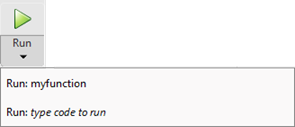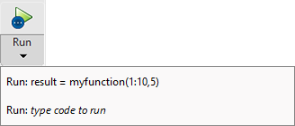Main Content

## Configure the Run Button for Functions

Functions are program files that accept inputs and return outputs. To run functions that require input argument values or any other additional setup from the Editor, configure thebutton.

To configure the button in the Editor, clickand add one or more run commands.

For example:

1. Create the function `myfunction.m` that performs a calculation using the inputs `x` and `y` and stores the results in `z`.

```function z = myfunction(x,y) z = x.^2 + y;```
2. Go to the Editor tab and click. MATLAB® displays the list of commands available for running the function.3. Click the last item in the list and replace the text type code to run with a call to the function including the required input arguments. For example, enter the text ```result = myfunction(1:10,5)``` to run `myfunction` with the input arguments `1:10` and `5`, and store the results in the variable `result`. MATLAB replaces the default command with the newly added command.To run multiple commands at once, enter the commands on the same line. For example, enter the text ```a = 1:10; b = 5; result = myfunction(a,b)``` to create the variables `a` and `b` and then call `myfunction` with `a` and `b` as the input arguments.

Note

If you define a run command that creates a variable with the same name as a variable in the base workspace, the run command variable overwrites the base workspace variable when you run that run command.

4. Click thebutton. MATLAB runs the function using the first run command in the list. For example, clickto run `myfunction` using the command `result = myfunction(1:10,5)`. MATLAB displays the result in the Command Window.

```result = 6 9 14 21 30 41 54 69 86 ```

To run the function using a different run command from the list, clickand select the desired command. When you select a run command from the list, it becomes the default for the button.

To edit or delete an existing run command, click, right-click the command, and then select Edit or Delete.

Note

Running live functions in the Live Editor using thebutton is only supported in MATLAB Online™. When you run a live function using thebutton, the output displays in the Command Window. To run a live function in an installed version of MATLAB, call the function from the Command Window or from a script or live script.

Download ebook